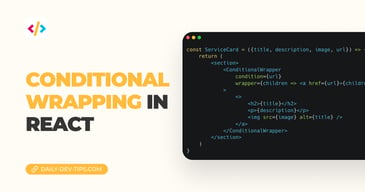Subscribe

# React basics: explaining the useState hook

••••••• + 27 devs liked it

✍️

How to use the useState hook in React

15 Oct, 2021 · 4 min read

Hooks are a superb way to store and manage state inside React components.

The useState hook is a super-easy way to maintain states efficiently.

## Declaring a new state permalink

To use the hook, we first need to import it from React like so:

``import React, { useState } from 'react'``

To use it, we can declare a new variable. Let's say we have a name variable.

``const [name, setName]  = useState(''); ``

Let's expand a bit on the above code piece:

• `name`: The variable name, we can display it to the user like so `{name}`.
• `setName`: The setter function as we can't modify the name variable directly.
• `useState('')`: The initial constructor, in this case, we set it to an empty string

You can set all kinds of default values some examples:

``````const [count, setCount] = useState(10);
const [show, setShow] = useState(false);
const [books, setBooks] = useState([]);
const [car, setCar] = useState({});``````

As you see, the naming convention for the set function has to be the same as the variable but prefixed with `set`.

As mentioned, it's super easy to use this state variable, as we can render it out or map it if it's an array.

``````<p>Your name must be {name}</p>

{books.map((book) => (
<Book />
))}``````

When it comes to updating the state, let's take the number example for a second.

``const [count, setCount] = useState(10);``

This will give us an initial value of 10. Let's then add a button that will invoke a function to add one each time we click it.

``````function App() {
const [count, setCount] = useState(10);

return (
<div className='App'>
<p>The count is {count}</p>
<button onClick={() => setCount(count + 1)}>Add one</button>
</div>
);
}

export default App;``````

This is the most basic example, and it works. However, it might give us some issues.

Let's change this a bit to demonstrate an issue we might have.

``````function App() {
const [count, setCount] = useState(10);

const addOne = () => {
setCount(count + 1);
setCount(count + 1);
};

return (
<div className='App'>
<p>The count is {count}</p>
</div>
);
}

export default App;``````

Same thing as before, but now we are using a function to add a new count. And actually, call it twice.

Should we expect to see the number go up by two, right? But this is not the case. Since React will take the initial value and has not been changed yet, it counts with that in the second call.

There is an easy way to fix this. The setter function comes with a previous value argument we can use like so:

``setCount((prevValue) => prevValue + 1);``

If we now change our code to look like this:

``````function App() {
const [count, setCount] = useState(10);

const addOne = () => {
setCount((prevValue) => prevValue + 1);
setCount((prevValue) => prevValue + 1);
};

return (
<div className='App'>
<p>The count is {count}</p>
</div>
);
}

export default App;``````

Now, we'll see the count increment by two each time we click!

I hope you had some fun learning about setState in React. I created this small playground for you to try out yourself.

See the Pen React Function Component Examples by Chris Bongers (@rebelchris) on CodePen.

Tweet this tip
••••••• + 27 devs liked it

### Conditional wrapping in React

11 Dec, 2022 · 4 min read### Have you tried React classnames?

26 Oct, 2022 · 2 min readJoin 2088 devs and subscribe to my newsletter

• 1000 articles written
• 2088 devs subscribed
• 529116 words written